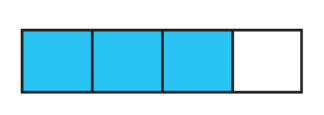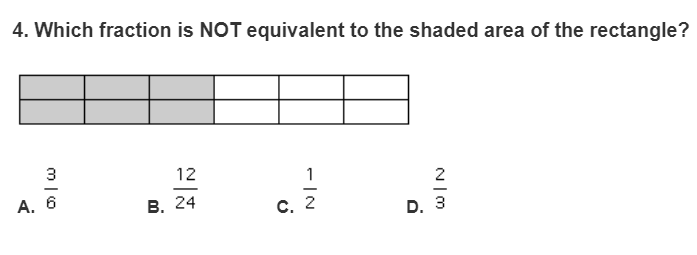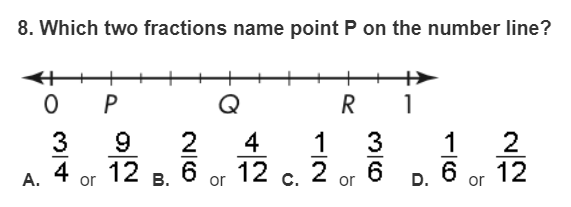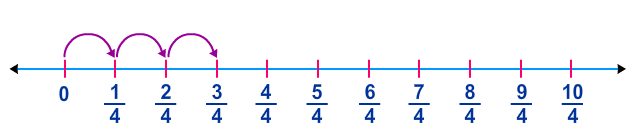Comparing Fractions
Equivalent Fractions
Subtracting Fractions
Unit Fractions
Identify the fraction
100

Which fraction is greater?

A) 3/8

B) 3/9

A) 3/8

100

1/2 = ? /10

5

100

1/4 + 3/4 =

4/4 or 1 whole

100

11/8 - 2/8 =

9/8 or 1 1/8

100

Decompose 7/8 as a SUM of unit fractions

1/8 + 1/8 + 1/8 + 1/8 + 1/8 + 1/8 + 1/8

100

Draw 2/4

200

Which fraction is smaller?

A) 5/8

B) 5/10

What is 5/10?

200

3/4 = 12/?

16

200

1/6 + 2/6 =

3/6

200

8/6 - ___ = 1/6

7/6

200

Decompose 9/8 as a Sum of unit fractions

1/8 +1/8 + 1/8 +1/8 + 1/8 + 1/8 + 1/8 + 1/8 +1/8

200

Draw 5/4

300

Is 9/10 greater or less than 1/2?

A) greater than

B) less than

A) What is greater than?

300

7/8 = 14/?

16

300What is this number line modeling?

5/9 + 2/9 = 7/9

300

3/4 - 2/4 =

1/4

300Write this as the sum of unit fractions.

1/4 + 1/4 + 1/4 = 3/4

300

Draw 1 1/4

400

Which fraction is greater?

A) 3/12

B) 3/8

B) What is  3/8?

400D. 2/3

400

4/11 + ____ = 1

7/11

400

1 - 1/2

1/2

400

Decompose 4/5 into two fractions

2/5 + 2/5 or 1/5 +3/5

400

Draw 7/12

500

Which fraction is smaller?

A) 5/9

D) 8/11

A) 5/9

500D. 1/6 and 2/12

500

Val's construction team was supposed to build a frame 7/10 meters long, but they ended up building the frame 2/10 too long. How long was the frame Val's team built?

9/10 meters long

500

Ruth had 7/8 block of cheese she used to make cheese dip. She used 2/8 block of cheese. How much does she have now?

5/8

500Using unit fractions, what is this number line modeling?

1/4 + 1/4 + 1/4 = 3/4

500

Draw 3 2/4

Click to zoom